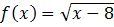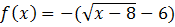### Sample Problem

Write an equation for the function that has the shape of f(x)=√x, but shifted eight units to the right, six units downward and reflected both in the x-axis and y-axis.

#### Solution

Start with the parent function f(x)=√x and apply the transformations in the order given.

Parent function:Shift eight units to the right:Shift six units downwards:Reflection in the x-axis:Reflection in the y-axis: